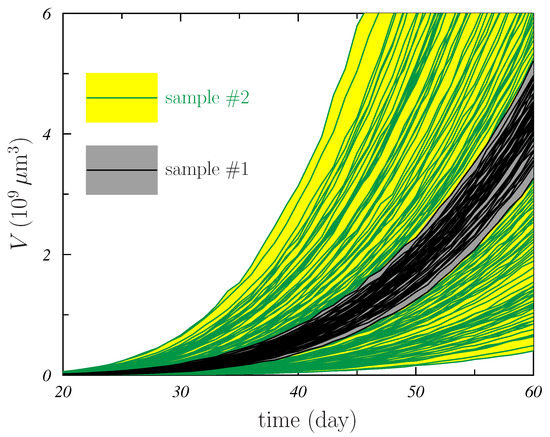Next Article in Journal
Review of Convective Heat Transfer Modelling in CFD Simulations of Fire-Driven Flows
Article

# Evaluation of Classical Mathematical Models of Tumor Growth Using an On-Lattice Agent-Based Monte Carlo Model †

1
Servicio de Oncología Radioterápica, Hospital Universitario Marqués de Valdecilla, E-39008 Santander, Spain
2
3
4
5
6
*
Author to whom correspondence should be addressed.
This work is part of the Ph.D. thesis developed by S. Ruiz-Arrebola within the Ph.D. program “Clinical Medicine and Public Health” at the University of Granada, Spain.
Appl. Sci. 2021, 11(11), 5241; https://doi.org/10.3390/app11115241
Received: 2 April 2021 / Revised: 21 May 2021 / Accepted: 28 May 2021 / Published: 4 June 2021
Purpose: To analyze the capabilities of different classical mathematical models to describe the growth of multicellular spheroids simulated with an on-lattice agent-based Monte Carlo model that has already been validated. Methods: The exponential, Gompertz, logistic, potential, and Bertalanffy models have been fitted in different situations to volume data generated with a Monte Carlo agent-based model that simulates the spheroid growth. Two samples of pseudo-data, obtained by assuming different variability in the simulation parameters, were considered. The mathematical models were fitted to the whole growth curves and also to parts of them, thus permitting to analyze the predictive power (both prospective and retrospective) of the models. Results: The consideration of the data obtained with a larger variability of the simulation parameters increases the width of the ${\chi }^{2}$ distributions obtained in the fits. The Gompertz model provided the best fits to the whole growth curves, yielding an average value of the ${\chi }^{2}$ per degree of freedom of 3.2, an order of magnitude smaller than those found for the other models. Gompertz and Bertalanffy models gave a similar retrospective prediction capability. In what refers to prospective prediction power, the Gompertz model showed by far the best performance. Conclusions: The classical mathematical models that have been analyzed show poor prediction capabilities to reproduce the MTS growth data not used to fit them. Within these poor results, the Gompertz model proves to be the one that better describes the growth data simulated. The simulation of the growth of tumors or multicellular spheroids permits to have follow-up periods longer than in the usual experimental studies and with a much larger number of samples: this has permitted performing the type of analysis presented here. View Full-Text
Show FiguresFigure 1

MDPI and ACS Style

Ruiz-Arrebola, S.; Guirado, D.; Villalobos, M.; Lallena, A.M. Evaluation of Classical Mathematical Models of Tumor Growth Using an On-Lattice Agent-Based Monte Carlo Model. Appl. Sci. 2021, 11, 5241. https://doi.org/10.3390/app11115241

AMA Style

Ruiz-Arrebola S, Guirado D, Villalobos M, Lallena AM. Evaluation of Classical Mathematical Models of Tumor Growth Using an On-Lattice Agent-Based Monte Carlo Model. Applied Sciences. 2021; 11(11):5241. https://doi.org/10.3390/app11115241

Chicago/Turabian Style

Ruiz-Arrebola, Samuel, Damián Guirado, Mercedes Villalobos, and Antonio M. Lallena. 2021. "Evaluation of Classical Mathematical Models of Tumor Growth Using an On-Lattice Agent-Based Monte Carlo Model" Applied Sciences 11, no. 11: 5241. https://doi.org/10.3390/app11115241

Find Other Styles
Note that from the first issue of 2016, MDPI journals use article numbers instead of page numbers. See further details here.

1